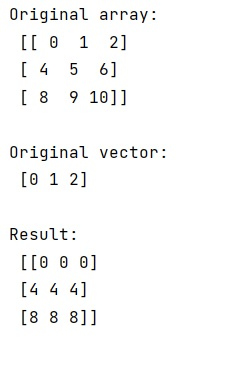# NumPy: Subtract every row of matrix by vector

Learn, how to subtract every row of a NumPy's matrix by a vector?
Submitted by Pranit Sharma, on January 24, 2023

NumPy is an abbreviated form of Numerical Python. It is used for different types of scientific operations in python. Numpy is a vast library in python which is used for almost every kind of scientific or mathematical operation. It is itself an array which is a collection of various methods and functions for processing the arrays.

## Subtracting every row of matrix by vector

Suppose that we are given a n x d matrix and a n x 1 vector and we need to subtract every row of this matrix by this vector.

If the trailing axes have the same dimension, NumPy will do our work. We will first create a n x d matrix followed by a n x 1 vector and simply subtract the matrix by this vector but if the trailing axes are not the same, we need to find the transpose of the matrix first then we will subtract the vector from it and again we need to find the transpose of the whole result.

Let us understand with the help of an example,

## Python code to subtract every row of matrix by vector

```# Import numpy
import numpy as np

# Import math
import math

# Creating a numpy array
arr = np.array([[ 0 , 1 , 2 ],
[ 4 , 5 , 6 ],
[ 8 , 9 ,10 ]])

# Display original array
print("Original array:\n",arr,"\n")

# Creating a vector
v = np.arange(3)

# Display original vector
print("Original vector:\n",v,"\n")

# Subtracting vector from array
res = arr-v

# Display result
print("Result:\n",res,"\n")
```

Output: